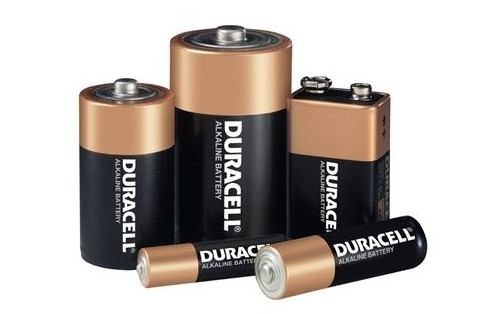# Batteries and Accumulators DemystifiedA battery is a device which stores chemical energy and which can convert it into electricity. There are two types of batteries: primary batteries (single use) and secondary batteries (rechargeable), also known as accumulators.

In this post, I will explain how to chose the right batteries for your projects and how to compute how long will that battery last.

# Primary Batteries (single use)

The primary batteries are based on irreversible electrochemical reactions, which makes them non-rechargeable. The primary advantage of these batteries is that they have a low self-discharge current and they’re used in applications which require long term energy storage.

The alkaline batteries are part of this category, they have a nominal cell voltage of 1.5 V and they offer an energy density of 85 Wh / kg, respectively 250 Wh / L (when related to the volume). Their self discharge rate is less that 0.3% / month.

# Secondary Batteries (rechargeable)

From this category, there are a few type of batteries worth mentioning: lead acid batteries, nickel metal hybrid (NiMh) batteries, lithium ion batteries (Li-Ion) and lithium ion polymer batteries (LiPo).

The lead acid batteries have a nominal cell voltage of 2.1 V and a quite low energy density (30-40 Wh/kg, respectively 60-75 Wh/L). They have a durability of 500-800 charge-discharge cycles and a time durability of 5-8 years (in automotive operating conditions).

The NiMh batteries have a nominal cell voltage of 1.2 V, an energy density of 30-80 Wh/kg, respectively 140-300 Wh/L, and a durability of 500-1000 cycles. The NiMh batteries have a high self-discharge current (about 30% / month).

The Li-Ion batteries have a nominal cell voltage of 3.6 V, an energy density of 150-250 Wh/kg (or 250-360 Wh/L), a durability of 1200-10000 cycles and a self discharge rate of 5-10% / month. The LiPo batteries have similar characteristics and a durability of 500-1000 cycles.

# How much energy does the battery actually store ?

The batteries are usually rated in mAh (for example 1000 mAh).  This rating actually measures the charge stored by the battery and it means that a 1000 mAh battery for example is capable to supply a current of 1000 mA for 1 h.

Knowing the nominal voltage and the rating of the battery, we can compute the amount of energy stored as follows:

$$E = V \cdot Q$$

Where:
$$E$$ is the energy that the battery can store (measured in joules; $$1 J = 1 Ws = 1 VAs$$)
$$V$$ is the nominal voltage of the battery
$$Q$$ is the charge that can be stored by the battery (measured in coulombs; $$1 C = 1 As$$)

For example, a battery with a rating of 1000 mAh can store the following charge:

$$Q = 1000 \cdot 10^{-3} \cdot 3600 As = 3600 C$$

If this battery has for example nominal voltage of 3.7 V, then it stores the following energy:

$$E = V \cdot Q = 3.7 \cdot 3600 J = 13320 J$$

# How long will the battery last ?

The amount of time that a battery lasts from a charge depends on the energy storage capacity of the battery and on the power consumption of the device that is powers.

You can compute the amount of time that a battery will last from a single charge as follows:

$$\large{t = \frac{E}{P}}$$

Where:
$$t$$ is the amount of time that the battery will last from a single charge (measured in seconds)
$$E$$ is the amount of energy that the battery can store (measured in joules)
$$P$$ is the power consumption of device which will be powered from the battery (measured in watts; $$1 W = 1 VA$$)

# What type of battery to choose ?

Overall, the LiPo batteries are usually the best option, considering the energy density, the price and the durability. They come in a many form factors, from a single LiPo battery cell to multi-cell packs. The LiPo chargers are readily available, and for one cell and two cell LiPo batteries, there are some chargers available even as small electronic modules.